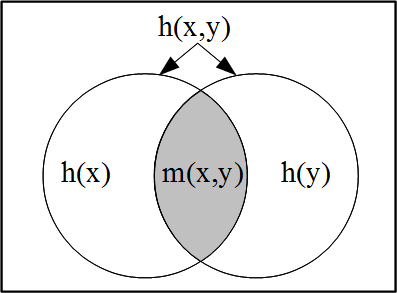# Introduction to Logical EntropyThe logical basis for information theory is the newly developed logic of partitions that is dual to the usual Boolean logic of subsets. The key concept is a “distinction” of a partition, an ordered pair of elements in distinct blocks of the partition. The logical concept of entropy based on partition logic is the normalized counting measure of the set of distinctions of a partition on a finite set–just as the usual logical notion of probability based on the Boolean logic of subsets is the normalized counting measure of the subsets (events). Thus logical entropy is a measure on the set of ordered pairs, and all the compound notions of entropy (join entropy, conditional entropy, and mutual information) arise in the usual way from the measure (e.g., the inclusion-exclusion principle)–just like the corresponding notions of probability. The usual Shannon entropy of a partition is developed by replacing the normalized count of distinctions (dits) by the average number of binary partitions (bits) necessary to make all the distinctions of the partition.